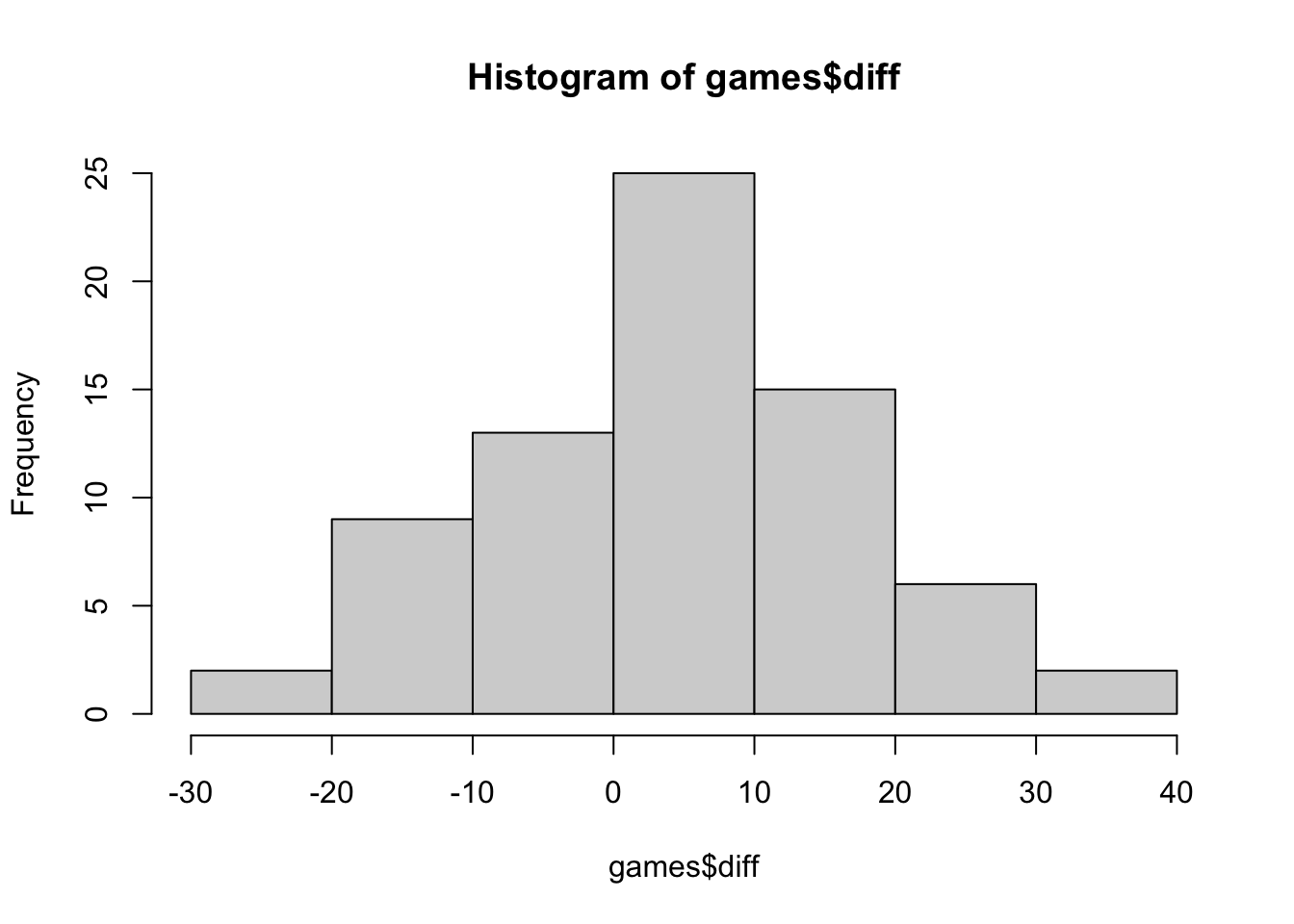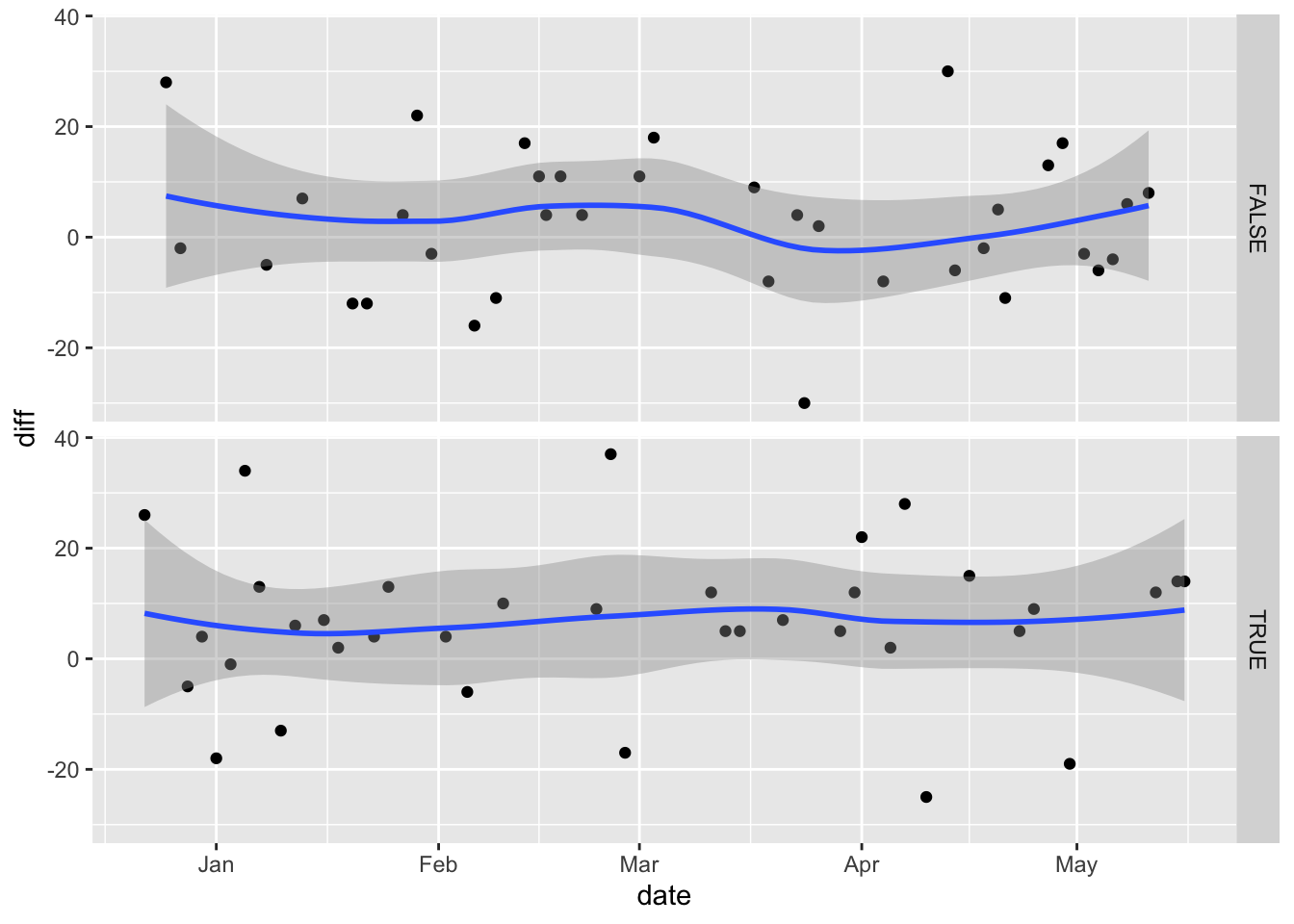In sceencast #2 I ask how the Nets performed in the regular NBA season depending on how many of their stars played.

## R Markdown

``````library(rvest)
library(tidyverse)
``````
``````irving <- "https://www.basketball-reference.com/players/i/irvinky01/gamelog/2021"

get_data <- function(player_url) {
tables <- html %>% html_nodes("table") %>% html_table()
df <- tables %>% data.frame()
return(df)
}
``````
``````df1 <- get_data(irving)
df2 <- get_data(harden)
df3 <- get_data(durant)
``````
``````nets_games_url <- 'https://www.basketball-reference.com/teams/BRK/2021_games.html'
tables <- html %>% html_nodes("table") %>% html_table()

nets_regular_season <- tables[] %>% data.frame()
nets_playoffs <- tables[] %>% data.frame()

games <- nets_regular_season %>% select(G, Date, Var.6, Opponent, Var.8, Tm, Opp)
names(games) <- c("G", "Date", "Home", "Opponent", "WinLoss", "Team", "Opp")
``````
``````games\$date <- apply(games, 1, FUN = function(x) {substr(x[c("Date")], 6, 20) })
games\$date <- as.Date(games\$date, c("%B %d, %Y"))

glimpse(games)
``````
``````## Rows: 75
## Columns: 8
## \$ G        <chr> "1", "2", "3", "4", "5", "6", "7", "8", "9", "10", "11", "12"…
## \$ Date     <chr> "Tue, Dec 22, 2020", "Fri, Dec 25, 2020", "Sun, Dec 27, 2020"…
## \$ Home     <chr> "", "@", "@", "", "", "", "", "", "", "@", "", "", "@", "", "…
## \$ Opponent <chr> "Golden State Warriors", "Boston Celtics", "Charlotte Hornets…
## \$ WinLoss  <chr> "W", "W", "L", "L", "W", "L", "L", "W", "W", "L", "L", "W", "…
## \$ Team     <chr> "125", "123", "104", "111", "145", "96", "122", "130", "122",…
## \$ Opp      <chr> "99", "95", "106", "116", "141", "114", "123", "96", "109", "…
## \$ date     <date> 2020-12-22, 2020-12-25, 2020-12-27, 2020-12-28, 2020-12-30, …
``````
``````games\$Team <- as.integer(games\$Team)
games\$Opp <- as.integer(games\$Opp)
games\$diff <- games\$Team - games\$Opp
hist(games\$diff)
````````````games\$home <- games\$Home != '@'
table(games\$home)
``````
``````##
## FALSE  TRUE
##    36    39
``````
``````games %>% ggplot(.) + geom_point(aes(y=diff, x=date)) + facet_grid(home ~ .) + geom_smooth(aes(x=date, y=diff))
````````````irving <- df1 %>% select(Rk, G)
names(irving) <- c("Rk", "Irving")

harden <- df2 %>% select(Rk, G)
names(harden) <- c("Rk", "Harden")

durant <- df3 %>% select(Rk, G)
names(durant) <- c("Rk", "Durant")
``````
``````m <- irving %>% left_join(harden, by=c("Rk"="Rk"))
m2 <- m %>% left_join(durant, by=c("Rk"="Rk"))
data <- m2 %>% filter(Rk != "Rk")
nrow(data)
``````
``````##  72
``````
``````data\$Irving <- ifelse(is.na(data\$Irving), "", data\$Irving)
data\$Harden <- ifelse(is.na(data\$Harden), "", data\$Harden)
data\$Durant <- ifelse(is.na(data\$Durant), "", data\$Durant)

data\$Irving <- data\$Irving != ""
data\$Harden <- data\$Harden != ""
data\$Durant <- data\$Durant != ""

data\$i <- ifelse(data\$Irving, "I", "")
data\$h <- ifelse(data\$Harden, "H", "")
data\$d <- ifelse(data\$Durant, "D", "")

data <- data %>% mutate(N_stars = Irving + Harden + Durant)

data <- data %>% mutate(star_combo = paste(i, h, d, sep=""))

dfm <- games %>% left_join(data, by=c("G"="Rk"))

dfm %>% ggplot(.) + geom_point(aes(y=diff, x=date)) + facet_wrap(. ~ N_stars) + geom_smooth(aes(x=date, y=diff))
``````